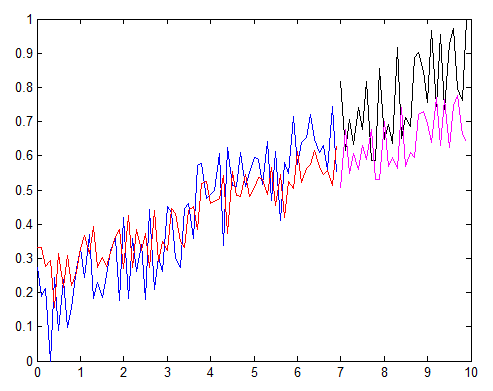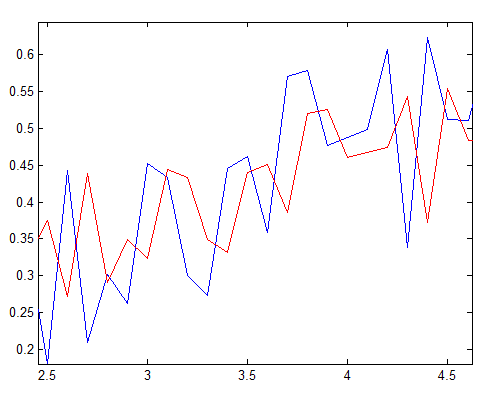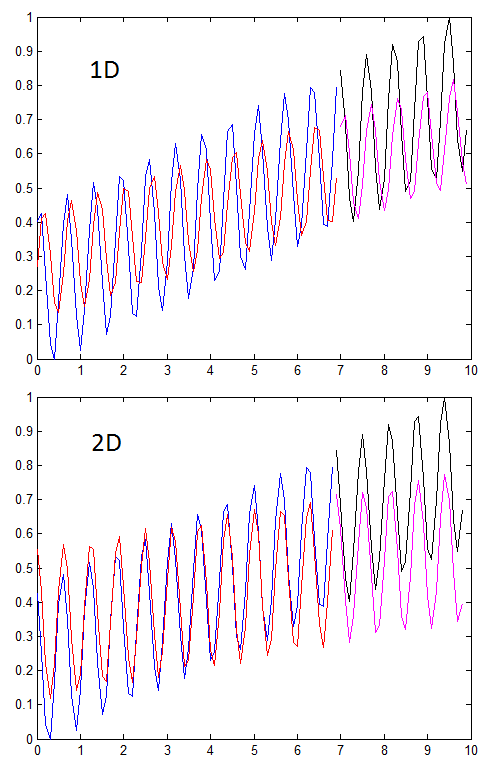### Archive

Archive for the ‘matlab’ Category

## Projection of a NIRS channel on a brain surface

April 11th, 2016

When analyzing the data in our concurrent NIRS-fMRI study, we are particularly interested in how the NIRS signals were correlated to the fMRI signal. To answer the question we need to create an ROI (region of interest) in brain which is directly underneath the NIRS channel (which is on the skull). So a projection of the NIRS channel on the brain surface is necessary.

It might be easy if the brain were a perfect sphere, or at least doable if it is smooth. But brain surface is anything but smooth. What we did is:

1. Create a brain surface mask. This is easy using BET (http://fsl.fmrib.ox.ac.uk/fsl/fslwiki/BET)
2. Loop over all voxels on the surface, calculate the distance between the NIRS channel (point A) and the voxel
3. Find the voxel which is closest to the NIRS channel (It is point B in the figure below)Projection of NIRS channel on brain surface

Xu Cui Categories: Tags:

## T value of a single subject

March 14th, 2016

I usually report a group-level T-test image in the final publications or presentations. But in the early stage of a project when only one or a few subjects have been scanned, I often need to report an activation map for an individual subject after general linear model analysis (GLM). I could show a beta image or activation image, but a T-image is sometimes desired. The question is how do I calculate the T-value of a contrast for a single subject.

I will use the following example to show how it is calculated. Assume we have two conditions, beep and flash, and the brain signal is saved in y. Based on the timing of beep and flash, we have an independent variable called x with two columns. The 1st column is for beep, the 2nd for flash.

```x=rand(10,2);
y=x(:,1)*2 + x(:,2)*3 + 0.2 + rand(size(x(:,1)))/5;
[a,b,c] = glmfit(x,y);
```

Now you can easily find the beta value, the T value, and the p-value of each individual condition:

```beta = a(2:end)'
T = c.t(2:end)'
p = c.p(2:end)'
```

What about the contrast between beep and flash? Well, it’s a bit more complicated. First, we need to find the covariance matrix of the betas.

```covb = c.covb(2:end, 2:end);
```

Then we define our contrast vector, in this case it is simply contrast = [1 -1]. The variance of contrast (beep-flash) is

```V = contrast  * covb * contrast';
```

The T-value of the contrast is

```T = (beta * contrast') / sqrt(V) * sqrt(length(x));
```

Note: you might be tempted to use the two-sample T formula to calculate the T-value (i.e. finding the variance of beta1, variance of beta2, and then calculate). It is not the correct way. We have to consider the case where the two conditions are correlated. Using the covariance matrix above is the right way.

Xu Cui Categories: Tags:

## Finger tapping task MatLab script

February 29th, 2016

Finger tapping is probably the most used task in brain imaging studies (fMRI and NIRS). The task is simple and elicits robust brain signal in the motor cortex. We always use it to test new devices or develop a new method.

If you want to control your NIRS device (say Hitachi ETG 4000) to start/end, please refer http://www.alivelearn.net/?p=1260

If you want to see how blood flow increases in brain motor cortex during finger tapping, check out
http://www.alivelearn.net/?p=1686

Xu Cui Categories: Tags:

## xjView 8.13 released. Allow to change the minimum of colorbar range

November 9th, 2015

xjView 8.13 is released with the following new features:

1. Allow to change the minimum value of the color bar range. This will enable you to do
1. Create a symmetric color bar from negative to positive. For example, from -8 to 8
2. Use cold color to show “negative” contrast. In this case, you simply set the max as 0, and min as a positive number such as 5
2. Allow to resize the xjView window

A short tutorial video: https://youtu.be/jixQvi0ccHc

Xu Cui Categories: Tags:

## NIRS hyperscanning data analysis (4) coherence analysis

November 2nd, 2015

How do we analyze hyperscanning data to find out the relationship between two brains? Obviously correlation is the first thought. However, raw correlation analysis might be susceptible to noise in the data (e.g. physiological noise such as heart beating, or motion noise). Wavelet coherence is a great way to find correlation between two signals - at the same time not affected by physiological noises. To understand wavelet coherence, you might want to read:

Let’s look at the data of a pair of subjects (please click the image to zoom):

There are 22 channels in total in each participant. Each small plot in the figure above is for one channel. The plots are arranged following the real position of the channel on the participant’s head. How did I arrange them? I cut and paste and arrange in PowerPoint :) You can imagine it took me some time. I wish there is a handy tool.

By looking at this figure, a pattern pops out - there is a band in the period 6.4s in multiple channels. Maybe this band (increase of coherence) is a signature of collaboration. To quantify this, we average the coherence value around the band (between periods 3.2 and 12.8s in the task block, and the rest period in between):

```[Rsq,period,scale,coi,sig95] = wtc(signal1,signal2,'mcc',0, 'ms',128);
period32 = find(period>32);
period32 = period32(1);
b1 = mean(mean(Rsq(period32:end, marktime(1):marktime(2))));
bi = mean(mean(Rsq(period32:end, marktime(2):marktime(3))));
b2 = mean(mean(Rsq(period32:end, marktime(3):marktime(4))));```

Now we get a 3 values for each channel. I normally define “coherence increase” as the average of the coherence during task minus the coherence in rest. So CI = (b1+b2)/2 - bi

For each channel in each subject pair, we got a single value (CI). Then for each channel, we can do a group analysis using T-test. After we get all the T-values for all channels, then we can plot the map using plotTopoMap

This is basic analysis. You can go much deeper from here. Below are some examples:

1. Is there a learning effect? i.e. is the performance during the 2nd block better than the 1st block? Is the coherence increase higher than the 1st block?
2. Does coherence increase in the collaboration block differ from other blocks (such as competition, independent blocks)?
3. Does the phase of the coherence carry any information?
Xu Cui Categories: Tags:

## How to label each point in MatLab plot?

April 27th, 2015

How to label each data point in a MatLab plot, like the following figure?

MatLab code:

```x = [1:10];
y = x + rand(1,10);

figure('color','w'); plot(x,y,'o');
a = [1:10]'; b = num2str(a); c = cellstr(b);
dx = 0.1; dy = 0.1;
text(x+dx, y+dy, c);```

It also works on 3D plot:

Xu Cui Categories: matlab Tags:

## SVM regression on time series, is there a lag?

March 23rd, 2015

It would be nice if we can predict the future. For example, give the following time series, can we predict the next point?Let’s use SVM regression, which is said to be powerful. We use the immediate past data point as the predictor. We train our model with the first 70% of data. Blue and Black are actual data, and Red and Pink are predicted data.The prediction in general matches the trend. But if you look closely, you see that the predicted data is always lagging the actual data by one time step. See a zoom in below.Why does this lag come from?

Let’s plot the predictor and the predicted (i.e. the current data point vs the next data point):

It took me a few hours to think about this. Well, the reason turns out to be simple. It’s because our SVM model is too simple (only taking the last data point as predictor): if a data has a increasing trend, then the SVM model, which only consider the immediate history, will give a high predicted value if the current data value is high, a low value if the current data value is low. As a consequence, the predicted value is actually more similar to the current value - and that gives a lag if compared to the actual data.

To reduce the lag, you can build a more powerful SVM model - say use the past 2 data points as the predictor. It will make a more reliable prediction - if the data is not random. See below comparison: you can easily see the lag is much smaller.Source code can be downloaded here test_svr. Part of the source code is adapted from http://stackoverflow.com/questions/18300270/lag-in-time-series-regression-using-libsvm

Xu Cui Categories: Tags:

## Use MatLab to move and click mouse, to press keyboard

January 27th, 2014

We have an interesting challenge in one of our projects. In our neuroimaging experiment, we need the participant to play a computer game while his brain is scanned (using a NIRS device ETG 4000 in this case). As you can imagine, we need to start the computer game and brain data collection at the same time to make sure the behavior data and neuroimaging data are synchronized. What we usually do is to write some code to start ETG 4000 programmaticly inside the game program; but we can not do it this time because this computer game is developed by others and we can’t inject code into it.

What we want to achieve, simply put, is to click the “Go” button of the game at the same time when we start ETG 4000.

Fortunately there is a solution. We can write a MatLab program to simulate mouse movement and click. Below is the matlab code which will automatically move the mouse to point (640,640) and click it after 5s. If your computer game program requires keyboard input, the code below also contains a snippet for that.

```import java.awt.Robot;
import java.awt.event.*;
robot = Robot;

% 5s later, move the mouse to point (640,640) where the 'go' button is,
% then click it.
pause(5);
robot.mouseMove(640, 640);
% fill in the code to start ETG 4000 here

% 5s later, press key SHIFT and W at the same time
%pause(5);
robot.keyPress(java.awt.event.KeyEvent.VK_SHIFT)
robot.keyPress(java.awt.event.KeyEvent.VK_W)
robot.keyRelease(java.awt.event.KeyEvent.VK_W)
robot.keyRelease(java.awt.event.KeyEvent.VK_SHIFT)```

As you can see in the following short screen shot, after we run the matlab program (called testmouse), 5s later the mouse moves to (640,640) and clicks, then key SHIFT+W is pressed.

Xu Cui Categories: Tags:

## Brain surface template

August 19th, 2013

Sometimes we need to work on the surface of a brain. Here I create a surface mask based on the standard avg152T1 image (MNI space) for you to download.

Visualized in xjview, you can see the mask in the yellow curved lines. It’s actually a surface. The background image is the standard avg152T1 image.

Xu Cui Categories: Tags:

## Handy programs to visualize NIRS data (6): plotAverage

July 15th, 2013

This is the 6th post of the series: Handy programs to visualize NIRS data

When we do an experiment, we often repeat an event (or block) for a few times. For example, in a typical finger tapping task, we ask the participants to do a finger tapping for 20s, then rest for 20s, then repeat the whole tap-rest paradigm for 10 time.

After we extract the NIRS time courses, we often need to know the average of the signal over all repetitions of the event. In the finger tapping experiment, we want to know the average signal across 10 blocks of finger tapping.

What we need to do is:

1. Know the timing of each block (of course!)
2. cut the NIRS signal into pieces. The starting points should be a few seconds before the onset timing of the experiment block; and the ending points should be a few seconds after the offset of the experiment block.
3. Align the pieces and average
4. Also calculate the standard deviation (or error) of the average
5. plot
The attached cuixuNIRSretrieve.m and plotAverage can automatically do the above steps. Below is a plot done by plotAverage. In this example, there are two types of events, fs and fb. Each was repeated 10 times. The plot below plot the average of each of the event, and also the standard deviation (the shaded area). The vertical grey bar is the onset timing of the events. From the plot we can see that fb elicits a bigger response than fs does.
You can download the two scripts at:
Xu Cui Categories: Tags: